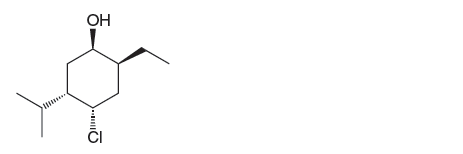Problem: Consider the following tetra-substituted cyclohexane: (b) Determine which conformation is more stable.

FREE Expert Solution
96% (366 ratings)
Problem Details

Consider the following tetra-substituted cyclohexane:

(b) Determine which conformation is more stable.What scientific concept do you need to know in order to solve this problem?

Our tutors have indicated that to solve this problem you will need to apply the Equatorial Preference concept. You can view video lessons to learn Equatorial Preference Or if you need more Equatorial Preference practice, you can also practice Equatorial Preference practice problems .

What is the difficulty of this problem?

Our tutors rated the difficulty of Consider the following tetra-substituted cyclohexane: (b) D... as low difficulty.

How long does this problem take to solve?

Our expert Organic tutor, Chris took 5 minutes to solve this problem. You can follow their steps in the video explanation above.

What professor is this problem relevant for?

Based on our data, we think this problem is relevant for Professor Hyvl's class at HAWAII.

What textbook is this problem found in?

Our data indicates that this problem or a close variation was asked in . You can also practice practice problems .